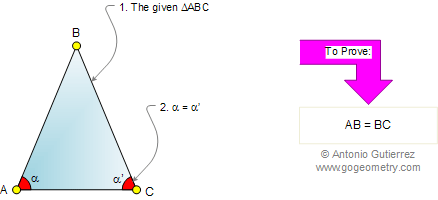Euclid's Elements Book I, Proposition 6:
If in a triangle two angles be equal to one another, the sides which subtend the equal angles will also be equal to one another.

Let ABC be a triangle having the angle BAC equal to the angle ACB;

I say that the side AB is also equal to the side BC. "Proof by contradiction," also called reductio ad absurdum.Geometrical conversion

Proposition I.6 is the geometrical, but not the logical, converse of proposition I.5.

The Elements: Books I-XIII

Euclid's Elements is the oldest mathematical and geometric treatise consisting of 13 books written by Euclid in Alexandria c. 300 BC. It is a collection of definitions, postulates, axioms, 467 propositions (theorems and constructions), and mathematical proofs of the propositions.

 The Thirteen Books of the Elements by Euclid